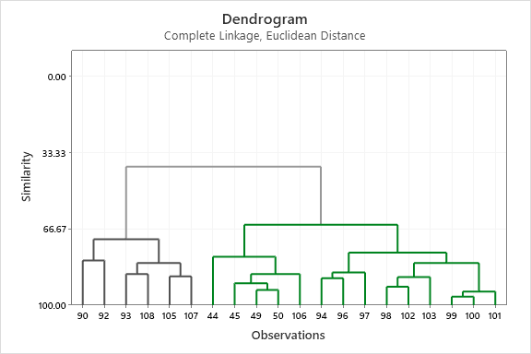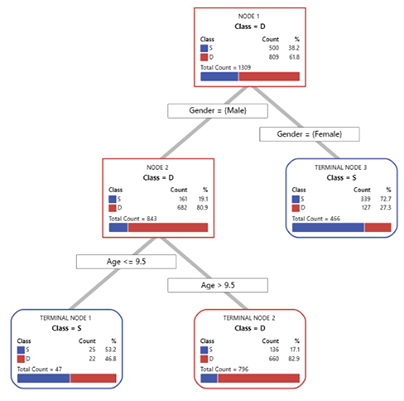# Training for Predictive Analytics## Training Courses

Our Predictive Analytics series is for professionals working in any industry doing predictive modeling. The course materials include examples with metrics such as payment performance, time, revenue, volume and quality grade.FUNDAMENTALS OF ANALYTICS

In this foundational course, you will learn to minimize the time required for data analysis with Minitab. We will cover how to import data, develop sound statistical approaches to exploring data, create and interpret compelling visualizations, and export results. You will discover how to automate your Minitab analysis with minimal user input, and that means saving time! We will analyze a variety of real-world data sets so you can learn how to align your applications with the right analytics tool and interpret the statistical output. Plus, you will learn the fundamentals of important statistical concepts, such as hypothesis testing and confidence intervals.

This course places a strong emphasis on making sound decisions based upon the practical application of statistical techniques commonly used in business, manufacturing, and transactional processes.

Topics include:

• Importing and Formatting Data
• Exec Macros
• Bar Charts
• Histograms
• Boxplots
• Pareto Charts
• Scatterplots
• Measures of Location and Variation
• t-Tests
• Test for Equal Variance
• Power and Sample Size

Prerequisites: None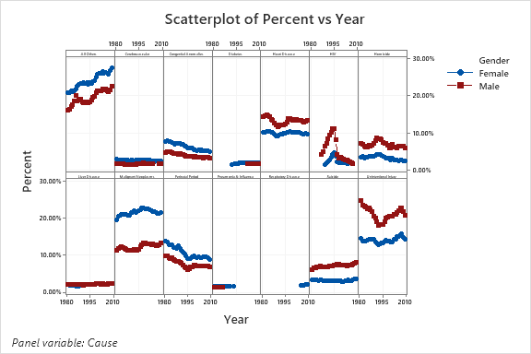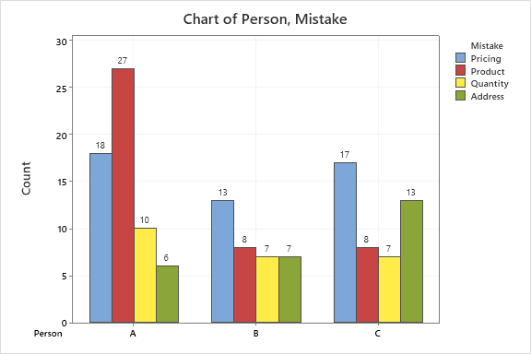REGRESSION MODELING AND FORECASTING

Ready to continue building on the fundamental statistical analysis concepts taught in the Fundamentals of Analytics? This course teaches you how to explore and describe relationships between variables with statistical modeling tools. You will discover and describe features in data related to the effect and impact of time, and how to forecast future behavior.

This course explains how to find and quantify the effect that input variables have on the probability of a critical event occurring. With hands-on examples, you’ll learn how modeling tools can help reveal key inputs and sources of variation in your data.

Topics include:

• Scatterplots
• Correlation
• Simple Linear Regression
• Time Series Tools, including Exponential Smoothing
• Trend Analysis
• Decomposition
• Multiple and Stepwise Regression
• Binary Logistic Regression
• Regression with Validation

Prerequisite: Fundamentals of Analytics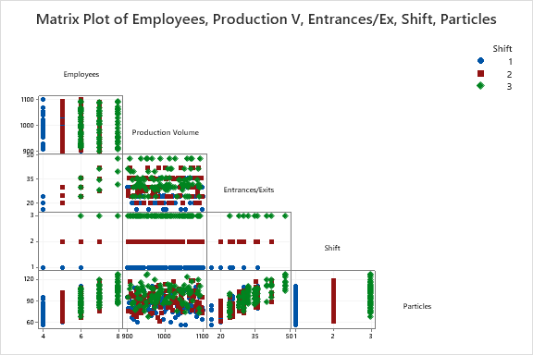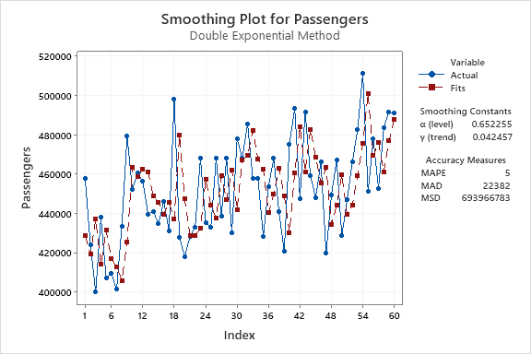MACHINE LEARNING

This course will help you expand your data analysis skills with real-world problem examples to teach you how to explore and describe relationships between variables. You will learn to use supervised machine learning techniques, such as CART®, to analyze patterns found in historical data, which can help you gain better insights, identify potential risks, seek out improvement opportunities, and make predictions about the future.

Use unsupervised machine learning tools, such as Clustering, to detect natural partitions in the data and group observations or variables into homogenous sets. Plus, reduce the dimensionality of data by transforming the original data into a set of uncorrelated variables.

Topics Include:

• Discriminant Analysis
• Test Set Validation
• K-fold Validation
• CART® Classification
• Correlation
• CART® Regression
• Cluster Analysis

Prerequisites: Fundamentals of Analytics, Regression Modeling and Forecasting